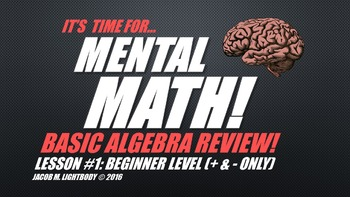# Mental Math: Basic Algebra Set 1Subject
Resource Type
Format
Zip (11 MB|4 PPTs)
\$2.00
\$2.00

### Description

This product offers four PowerPoint review lessons for practicing "mental math" with basic algebra problems. This is the first algebra installment in the Mental Math series. It addresses algebra problems involving addition and subtraction with a single variable. Supplemental lessons will follow that will address multiplication and division. Each PowerPoint contains ten problems.

PowerPoint 1: Beginner Level Practice (Sample Problem: 3 = 9 - n)

PowerPoint 2: Intermediate Level Practice (Sample Problem: 7 + n = 23)

PowerPoint 3: Intermediate Level Practice (Sample Problem: n + 8 = 36)

PowerPoint 4: Challenge Level Practice (Sample Problem: 16 – 9 = n + 1)

A preview file has been provided.

A collection of all current Mental Math sets (with twenty PowerPoints in all) is available as a bundle for \$4 by clicking this link.

The bundle includes:

Mental Math Subtraction (4 PPTs)
Mental Math Division & Remainders (4 PPTs)
Mental Math Fractions, Decimals & Percents (4 PPTs)
Mental Math Basic Algebra (4 Addition & Subtraction PPTs)

Total Pages
4 PPTs
Included
Teaching Duration
N/A
Report this Resource to TpT
Reported resources will be reviewed by our team. Report this resource to let us know if this resource violates TpT’s content guidelines.